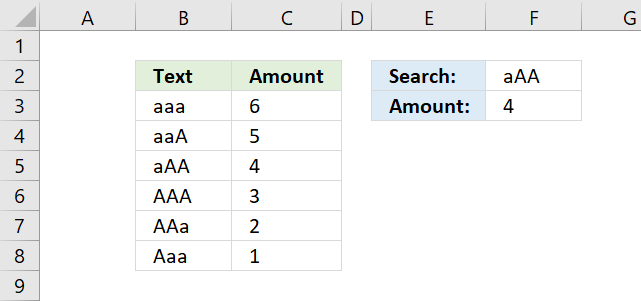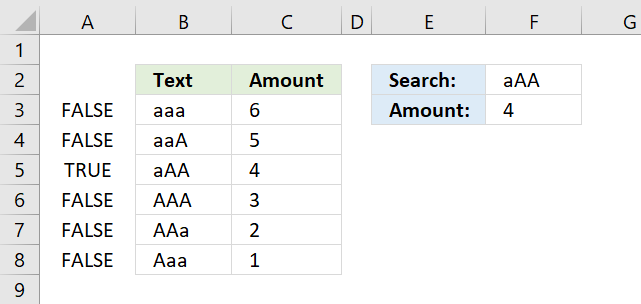Author: Oscar Cronquist Article last updated on November 15, 2018The picture above demonstrates a formula in cell F3 that allows you to look up a value in column B using the value in cell F2, also considering letter casing, then return the corresponding value from column C.

=INDEX(C3:C8,MATCH(TRUE,EXACT(F2,B3:B8),0))

The formula above is an array formula. To enter an array formula, type the formula in a cell then press and hold CTRL + SHIFT simultaneously, now press Enter once. Release all keys.

The formula bar now shows the formula enclosed with curly brackets telling you that you entered the formula successfully. Don't enter the curly brackets yourself.

If you prefer a regular formula, skip to "Alternative regular formula", below in this article.

### Explaining formula in cell F3

#### Step 1 - Compare lookup value with lookup column

The Exact function allows you to compare values, if they are exactly the same the EXACT function returns TRUE. Note, the function is case sensitive.

EXACT(F2,B3:B8)

becomes

EXACT("aAA",{"aaa"; "aaA"; "aAA"; "AAA"; "AAa"; "Aaa"})

and returns {FALSE; FALSE; TRUE; FALSE; FALSE; FALSE}.#### Step 2 - Identify the relative position of value TRUE in the array

The MATCH function finds a specific value in an array or cell range and returns its location, a number representing the position.

MATCH(TRUE,EXACT(F2,B3:B8),0)

becomes

MATCH(TRUE,{FALSE; FALSE; TRUE; FALSE; FALSE; FALSE},0)

and returns 3. TRUE is in the third position in the array.

#### Step 3 - Return corresponding value from column C

The INDEX function returns a value from an array or cell range based on the location. That is why the INDEX and MATCH function work so good together.

INDEX(C3:C8,MATCH(TRUE,EXACT(F2,B3:B8),0))

becomes

INDEX(C3:C8,3)

becomes

INDEX({6;5;4;3;2;1},3)

and returns 4 in cell F3.

### Alternative regular formula

=INDEX(C3:C8, MATCH(TRUE, INDEX(EXACT(F2, B3:B8), ), 0))